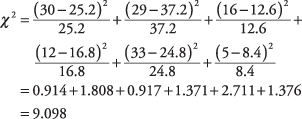## Chi-Square (X2)

The statistical procedures that we have reviewed thus far are appropriate only for numerical variables. The chi‐square2) test can be used to evaluate a relationship between two categorical variables. It is one example of a nonparametric test. Nonparametric tests are used when assumptions about normal distribution in the population cannot be met. These tests are less powerful than parametric tests.

Suppose that 125 children are shown three television commercials for breakfast cereal and are asked to pick which they liked best. The results are shown in Table 1.

You would like to know if the choice of favorite commercial was related to whether the child was a boy or a girl or if these two variables are independent. The totals in the margins will allow you to determine the overall probability of (1) liking commercial A, B, or C, regardless of gender, and (2) being either a boy or a girl, regardless of favorite commercial. If the two variables are independent, then you should be able to use these probabilities to predict approximately how many children should be in each cell. If the actual count is very different from the count that you would expect if the probabilities are independent, the two variables must be related.Consider the upper‐right cell of the table. The overall probability of a child in the sample being a boy is 75 ÷ 125 = 0.6. The overall probability of liking Commercial A is 42 ÷ 125 = 0.336. The multiplication rule states that the probability of both of two independent events occurring is the product of their two probabilities. Therefore, the probability of a child both being a boy and liking Commercial A is 0.6 × 0.336 = 0.202. The expected number of children in this cell, then, is 0.202 × 125 = 25.2.

There is a faster way of computing the expected count for each cell: Multiply the row total by the column total and divide by n. The expected count for the first cell is, therefore, (75 × 42) ÷ 125 = 25.2. If you perform this operation for each cell, you get the expected counts (in parentheses) shown in Table 2.Note that the expected counts properly add up to the row and column totals. You are now ready for the formula for χ 2, which compares each cell's actual count to its expected count:The formula describes an operation that is performed on each cell and which yields a number. When all the numbers are summed, the result is χ 2. Now, compute it for the six cells in the example:The larger χ 2, the more likely that the variables are related; note that the cells that contribute the most to the resulting statistic are those in which the expected count is very different from the actual count.

Chi‐square has a probability distribution, the critical values for which are listed in Table 4 in "Statistics Tables." As with the t‐distribution, χ 2 has a degrees‐of‐freedom parameter, the formula for which is

(number of rows – 1) × (number of columns – 1)